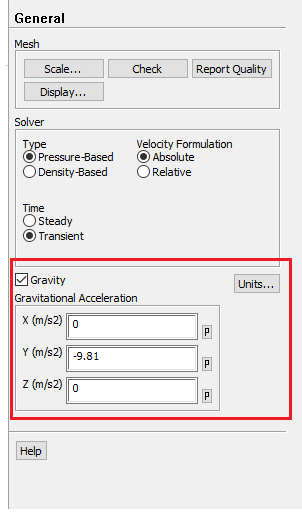## Fluids

#### In Natural Convection application : How can i fix the slope of duct for example

•AGAAMAZ
Subscriber
Hello everyone ;nIn Natural Convection application : How can i fix the slope of duct for example as (delta h) make difference in calculationnif i want the duct inclined 45 deg or 20 deg ... how can i do this ?nand is there a standard direction in ANSYS ?n
•peteroznewman
Subscriber
ArraynUse Gravity components to make down point at any angle you want.n
•AGAAMAZ
Subscriber
nthank you for your reply sirndo you mean form here , if yes could you please give me an examplenn
•peteroznewman
Subscriber
nIf you have a duct whose length lies along the X axis and cross-section is in the YZ plane, the gravity direction you show is for a horizontal duct.nIf you want a vertical duct, then you just type X = -9.81, Y = 0 and Z = 0.nIf you want a duct with a 30 degree upward slope along the X axis relative to horizontal, then nX = -9.81*sin(30) = -4.905nX = -9.81*cos(30) = -8.496nZ = 0n